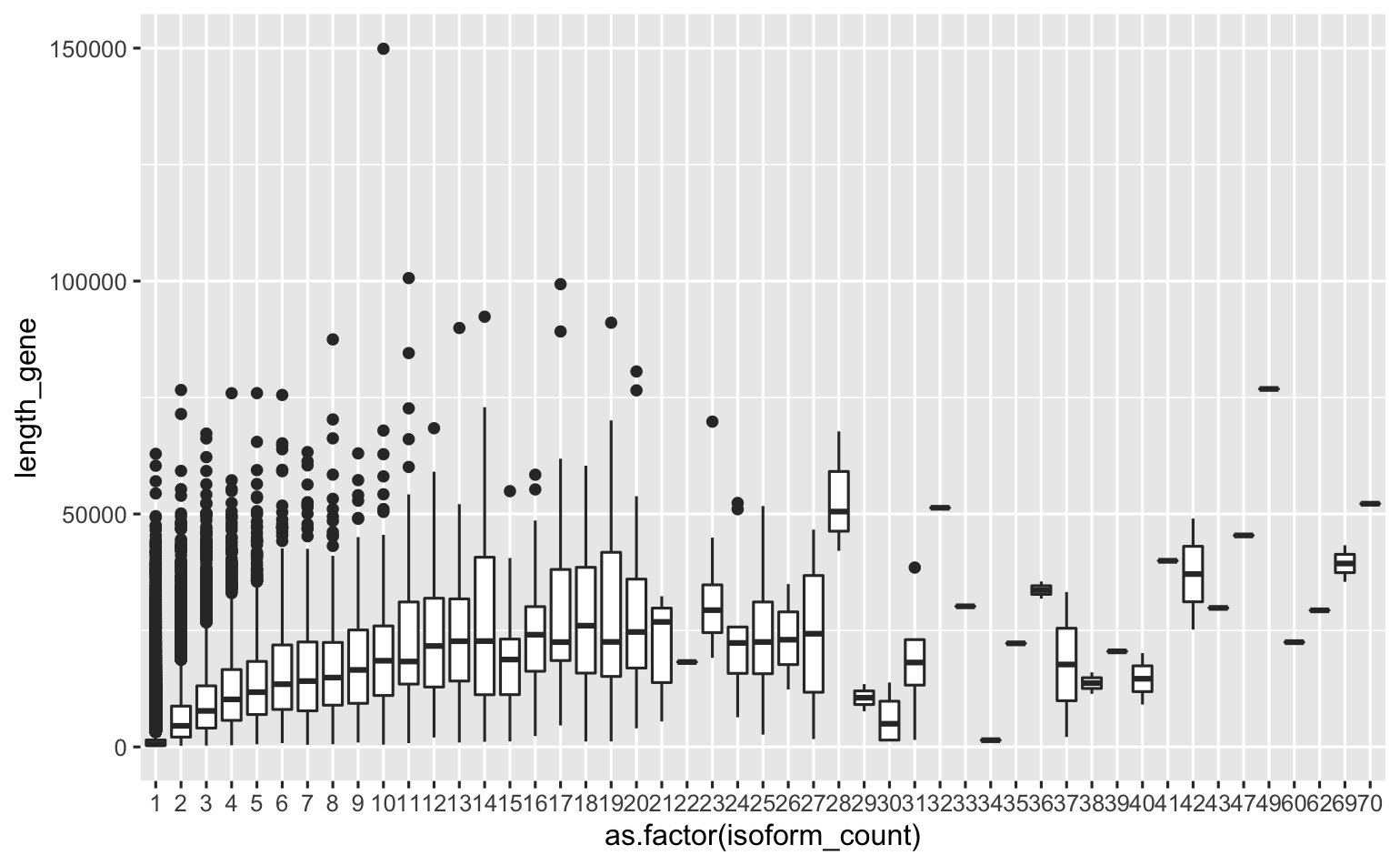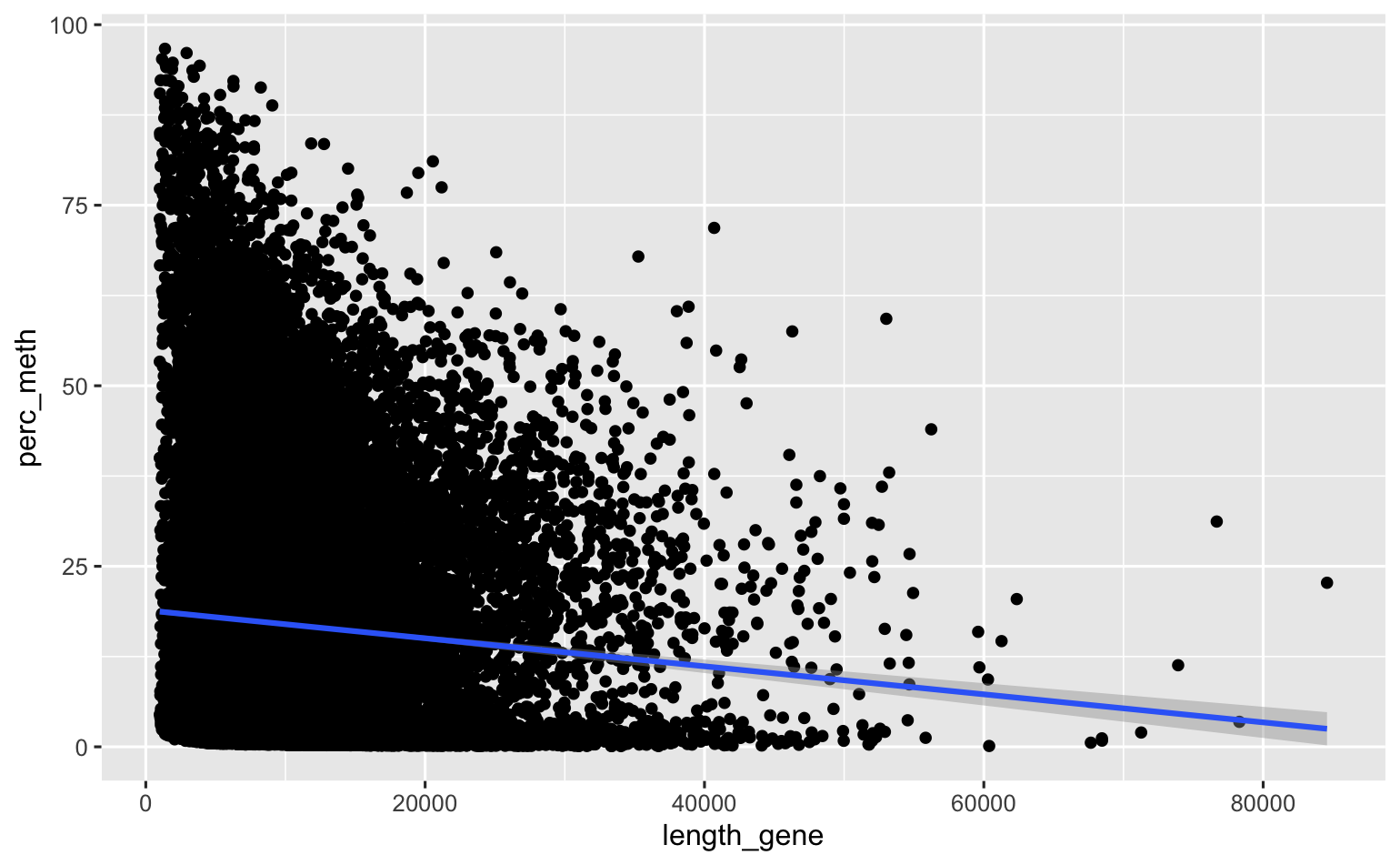# Oly methylation analysis, some general characteristic questions

I’m exploring the Oly MBD-Seq data to compare general methylation characteristics in O. lurda compared to other oysters. Here are a couple questions I investigated recently:

### Question: Does gene length ~ # isoforms?

If so, a CpG could be more likely since it’s longer. This could be a weird artifacts of transcriptome assembly could lead to genes having multiple isoforms - e.g. long. Here’s code from the 01c-Generating-meth-feature-files.Rmd notebook (where I produce the isoform feature files).

``````#ggplotly(
ASV.per.gene %>% mutate(length_gene=stop_gene-start_gene) %>%
ggplot() + geom_boxplot(aes(x=as.factor(isoform_count), y=length_gene))
#)
``````

#### My take: yes, gene length increases with isoform number for genes with ~1-15 isoforms. Then, there’s no relationship.### Question: Are longer genes more methylated?

Wang et al. 2014 found a positive association between gene length and methylation rate. Do I? Could that relate to the isoform relationship? Here I plot % of CpGs in a gene methylated, by gene length.

``````library(data.table)

left_join(
fread(here::here("analyses", "methylation-characteristics", "methylated-gene2kb.bed"), sep = "\t", select=c(5,8,9)) %>%
count(V5, V8, V9) %>%  setNames(c("contig_gene", "start_gene", "end_gene", "n_mCpG")),

count(V10, V13, V14) %>%  setNames(c("contig_gene", "start_gene", "end_gene", "n_CpG")),

by=c("contig_gene", "start_gene", "end_gene")) %>%

mutate(length_gene=end_gene-start_gene, perc_meth=100*(n_mCpG/n_CpG)) %>%
na.omit(perc_meth) %>%
ggplot(aes(x=length_gene, y=perc_meth)) + geom_point() +
geom_smooth(method="lm")
``````

#### My take: looks like a negative relationship. HOWEVER coverage is probably an issue here (longer genes less likely to be fully sequenced). Need to figure out how to do this in MethylKit, or how to only analyze genes with good coverage.Written on December 8, 2020